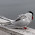## Tuesday, January 14, 2014

### Raster Calculations with numpy.where

The following may seem obvious to experienced users, but I did not find much documentation on the web on this:

With satellite images, one typically wants to do raster calculations. The easy way is to loop through the raster pixel by pixel, but this takes a long time with huge rasters. In this case, I want to calculate the precentage of sea ice. The daily percentage of the input "iceraster" is cumulatively added to the outputraster:

```    #Loop through all files to do calculation
for infile in filelist:

(infilepath, infilename) = os.path.split(infile)
print 'Processing ', infilename

#open the IceChart
icechart = gdal.Open(infile, gdalconst.GA_ReadOnly)
if infile is None:
print 'Could not open ', infilename
return

#get image size
rows = icechart.RasterYSize
cols = icechart.RasterXSize

#get the bands
outband = outraster.GetRasterBand(1)

#Read input raster into array

#Process the image by looping through the pixels
for i in range(rows):
for j in range(cols):
if iceraster[i,j] == 0:
outarray[i,j] = outarray[i,j] + (  0.0 / NumberOfDays)
elif iceraster[i,j] == 5:
outarray[i,j] = outarray[i,j] + (  5.0 / NumberOfDays)
elif iceraster[i,j] == 25:
outarray[i,j] = outarray[i,j] + ( 25.0 / NumberOfDays)
elif iceraster[i,j] == 55:
outarray[i,j] = outarray[i,j] + ( 55.0 / NumberOfDays)
elif iceraster[i,j] == 80:
outarray[i,j] = outarray[i,j] + ( 80.0 / NumberOfDays)
elif iceraster[i,j] == 95:
outarray[i,j] = outarray[i,j] + ( 95.0 / NumberOfDays)
elif iceraster[i,j] == 100:
outarray[i,j] = outarray[i,j] + (100.0 / NumberOfDays)

if iceraster[i,j] == 999:
outarray[i,j] = 999.0
```

The better way is to do calculations on the whole array/raster to use numpy.where. It is in a way similar to the band math-tool in some software packages. The syntax is:

``` numpy.where ( [condition], [if TRUE do this], [if FALSE do this] ) ```

Using this, the if-loop above can be rewritten like this (and does the calculation VERY much faster):

`        outarray = numpy.where((iceraster==0.0),(outarray + ( 0.0/NumberOfDays)), outarray)outarray = numpy.where((iceraster==5.0),(outarray + ( 5.0/NumberOfDays)), outarray)outarray = numpy.where((iceraster==25.0),(outarray + (25.0/NumberOfDays)), outarray)outarray = numpy.where((iceraster==55.0),(outarray + (55.0/NumberOfDays)), outarray)outarray = numpy.where((iceraster==80.0),(outarray + (80.0/NumberOfDays)), outarray)outarray = numpy.where((iceraster==95.0),(outarray + (95.0/NumberOfDays)), outarray)outarray = numpy.where((iceraster==100.0),(outarray + (100.0/NumberOfDays)), outarray)outarray = numpy.where((iceraster==999.0), 999.0 , outarray)`

The code above checks again the iceraster value and does the calculation, otherwise the output array is laft as it is.

The output is monthly ice percentage as seen here:

1.2.